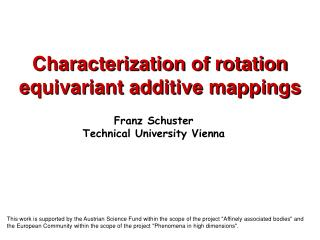DownloadDownload PresentationCharacterization of rotation equivariant additive mappings

# Characterization of rotation equivariant additive mappings

Télécharger la présentation## Characterization of rotation equivariant additive mappings

- - - - - - - - - - - - - - - - - - - - - - - - - - - E N D - - - - - - - - - - - - - - - - - - - - - - - - - - -
##### Presentation Transcript

1. Characterization of rotation equivariant additive mappings Franz Schuster Technical University Vienna This work is supported by the Austrian Science Fund within the scope of the project "Affinely associated bodies" and the European Community within the scope of the project "Phenomena in high dimensions".

2. (ii)  is equivariant with respect to SO(n) (K ) = (K )  SO(n) (iii)  is additive (K L) = (K) (L)K, LKn either Minkowski or Blaschke addition Definition: : Kn  Kn is a rotation equivariant additive map if (i)  is continuous

3. h(K,u) K h(K + L, . ) = h(K, . ) + h(L, . ) u - h(K,-u) o Sn – 1(K # L, . ) = Sn – 1(K, . ) + Sn – 1(L, . ) (K,) K Sn – 1(K,) = voln – 1((K, ))  Sn – 1 Minkowski addition: Blaschke addition:

4. Steiner point s: Kn IRn is a Minkowski endomorphism Projection operator  (K # L) = K + L : Kn  Kn is a Blaschke Minkowski homomorphism Examples:

5. : K2  K2 is a Minkowski endomorphism if and only if there is a weakly positive measure µ  M(S1) such that A measure is called weakly positive if it is nonnegative up to addition of a measure with density ux.u.  For n = 2 Minkowski endomorphisms are weakly monotone: K L and s(K) = s(L) = o  K  L Theorem [Schneider,74]:

6. : Kn  Kn is a weakly monotone Minkowski endomorphism if and only if there is a weakly positive zonal measure µ  M(S n – 1) such that h(K, . ) = h(K, . ) * µ. A measure is called zonal if it is SO(n – 1) invariant. : Kn  Kn is a Blaschke endomorphism if and only if there is a weakly positive zonal measure µ  M(S n – 1) such that Sn – 1(K, . ) = Sn – 1(K, . ) * µ. Theorem [Kiderlen,99]:

7. Theorem [F.S.,04]: If : Kn  Kn is a Blaschke Minkowski homomorphism there is a weakly positive zonal function g  C(S n – 1) such that h(K, . ) = Sn – 1(K, . ) *g. : Kn  Kn is a symmetric Blaschke Minkowski homomorphism if and only if there is a symmetric body of revolutionL  Kn such that h(K, . ) = Sn – 1(K, . ) *h(L, . ).   is a 'sum' of symmetric bodies of revolution

8. If : Kn Kn is a Blaschke Minkowski homomorphism there is a sequence of real numbers ck such that kh(K, . ) = ckkSn – 1(K, . )  KKn. k: M(Sn – 1)  Hkn is the orthogonal projection on the space Hkn of spherical harmonics of degree k Example [Goodey, Weil, 92]: Multiplier transformations:  Injectivity of  is equivalent to ck  0

9. ? "=" only if K is a ball "=" only for ellipsoids Lutwak‘s extension of Petty's conjecture: i = 0, …, n – 2 ? i = n – 2 Steiner formula for : Petty‘s conjectured projection inequality:

10. Theorem [F.S.,05]: "=" only for balls "=" only if n – 2K is a ball Conjecture: i = 0, …, n – 3 ? Steiner formula for B-M-homomorphisms: